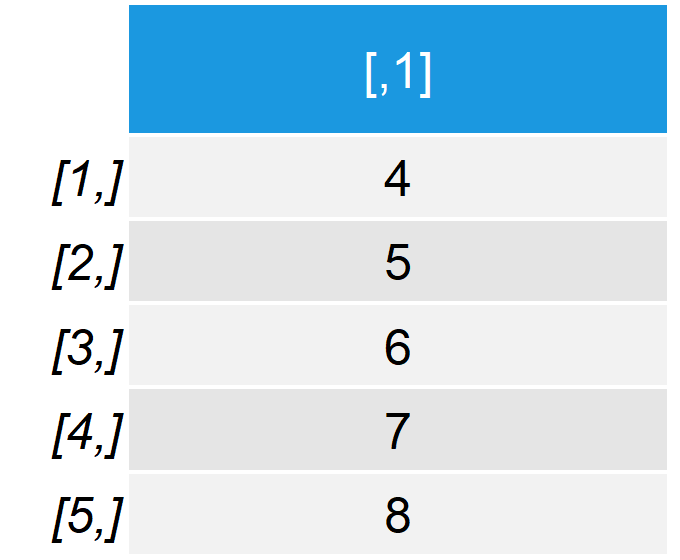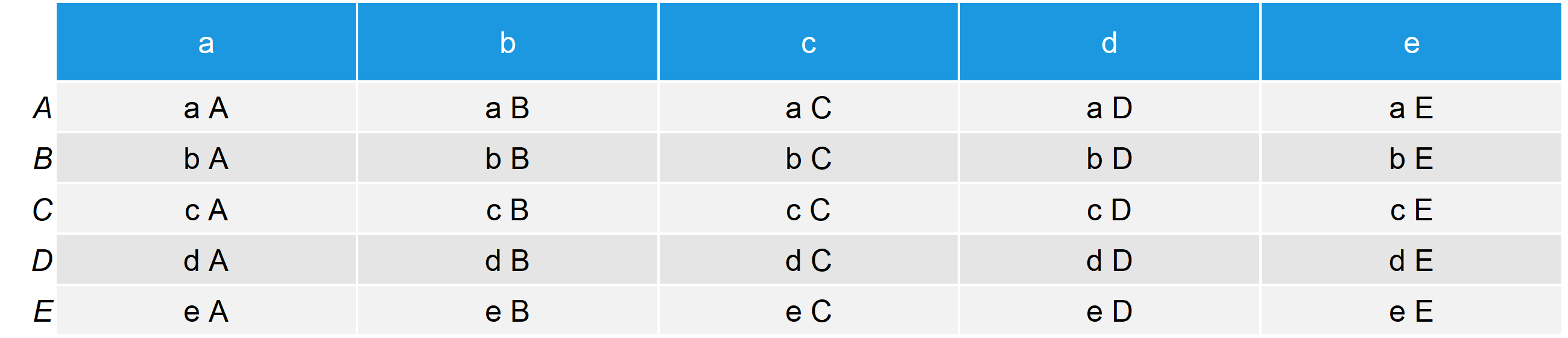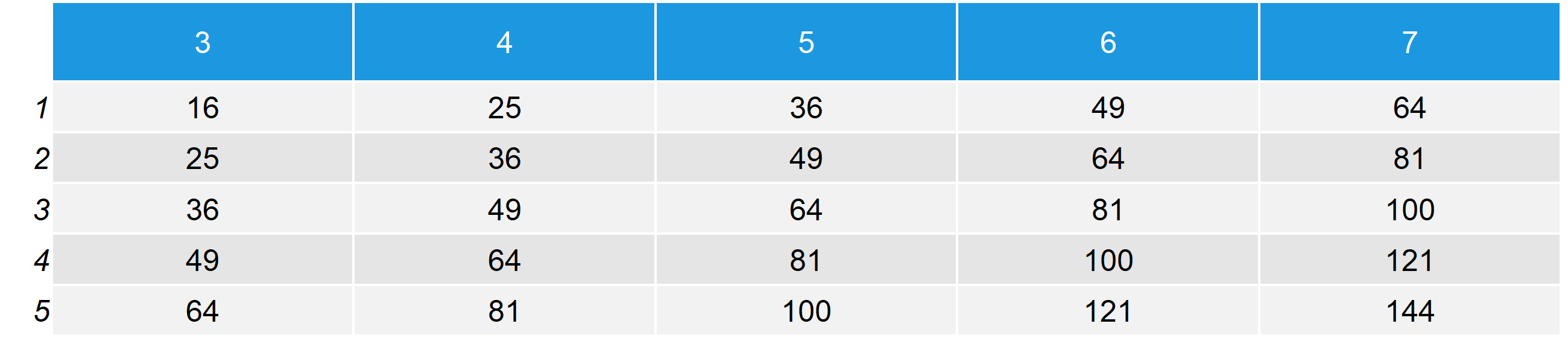# R outer Function | 4 Example Codes (Basic Application & User Defined)

Basic R Syntax:

`outer(x, y, "*")`

The R outer function applies a function to two arrays. The basic R code for the outer command is shown above.

In the following tutorial, I’m going to show you four examples for the usage of outer in R.

## Example 1: outer Function for Vector and Single Value

Probably the most basic usage of outer in R is the application to a numeric vector and a single value. Let’s create such data for the first example in R:

```x1 <- 1:5                                 # Create x vector
y1 <- 3                                   # Create y value```

Our example vector consists of the values 1 to 5 and as single value we are going to use the number 3.

We can apply the R outer function to this data with the following syntax:

```output1 <- outer(x1, y1, "+")             # Apply R outer function
output1                                   # Print output to RStudio console```Table 1: Output of First Example of outer() Function in R.

Table 1 shows the RStudio console output of the outer function. As you can see, the value 3 was added to each entries of our example vector, i.e. 1 + 3; 2 + 3; 3 + 3; 4 + 3; and 5 + 3.

In this example, I have used the + function, but we could use any other function we want.

That was easy, right? So let’s move on with some more advanced examples…

## Example 2: Outer Product of Two Vectors

In the second example, I’m going to show you how to apply outer in R to two numeric vectors (or arrays). First, let’s create some example data:

```x2 <- 1:5                                 # Create x vector (same as in Example 1)
y2 <- 3:7                                 # Create y vector```

Before I continue with the application of outer(), I’m going to show you a little trick, which will make the output much prettier. With the following R code, we can specify the name of the output matrix, which we will produce with the outer function in a second:

```names(x2) <- x2                           # Prepare row names of output matrix
names(y2) <- y2                           # Prepare column names of output matrix```

Now, let’s apply the outer R function:

```output2 <- outer(x2, y2, "+")             # Apply R outer function
output2                                   # Print output to RStudio console```Table 2: Output of Second Example of outer() Function in R.

We used exactly the same code as in Example 1, but this time with two vectors. As you can see, the outer command returns a matrix in which all combinations of the two vectors are stored.

For instance, in the cell of row 1 and column 1, we took the sum of 1 + 3 (i.e. the first entries of our first and second vector, respectively).

You can also see that the row and colnames of our output matrix are labelled with the values of our input vectors. That’s what we did with the little renaming trick I’ve shown you before.

Now you can go from cell to cell of the output matrix to see the result of each combination of our two vectors.

However, there is even more. Let’s continue with the next example…

## Example 3: Use outer for Different Data Types

So far, we have only used numeric values and vectors. However, the outer R command can also be used for other data types. In this example, I’ll show you how to use the outer command for characters.

Again, let’s begin with some new data:

```x3 <- letters[1:5]                        # Create x vector with characters
y3 <- LETTERS[1:5]                        # Create y vector with upper case```

As you can see, we created two character vectors, both consisting of the letters a, b, c, d, and e. For illustration, we use uppercase letters in case of the second vector.

We can now use exactly the same R syntax as in the previous examples. So first, let’s use our little trick in order to rename the column names of our output matrix…

```names(x3) <- x3                           # Prepare row names of output matrix
names(y3) <- y3                           # Prepare column names of output matrix```

…and then let’s apply the R outer function:

```output3 <- outer(x3, y3, "paste")         # Apply R outer function to characters
output3                                   # Print output to RStudio console```Table 3: Output of Third Example of outer() Function in R.

Same result as before, but this time with characters. Each possible combination of our two vectors is stored in the output matrix.

Note: This time we didn’t apply the + function to our two vectors (this would not be meaningful in case of characters). Instead, we used the paste function to concatenate the character values of our two vector strings.

So, with the next example we are moving on to the expert level. Let’s do this!

## Example 4: Apply outer to User Defined Custom Function

As you have seen in the examples before, outer can be applied to basically any function. So, why shouldn’t we create our own R function and apply outer to this function?

As in the previous examples, let’s start with some simple synthetic data:

```x4 <- 1:5                                 # Create x vector (same as in Example 1 & 2)
y4 <- 3:7                                 # Create y vector (same as in Example 2)```

For simplicity, I’m just using the same data as in Example 2. Now comes the important step – Let’s create our own function.

I’m not going to show you in this article how to specify functions in R yourself (this would blow up the tutorial too much). However, have a look HERE, if you want to learn more about that.

So, our user defined function looks as follows:

```custom_fun <- function(x, y) {            # Create custom function in R
z <- (x + y)^2
return(z)
}```

We are basically just adding our two values and take the sum to the power of 2. Of cause, you can make the function more complicated if you want.

Now, let’s use our typical R outer code. First, the little trick for the colnames…

```names(x4) <- x4                           # Prepare row names of output matrix
names(y4) <- y4                           # Prepare column names of output matrix```

…and then the outer R function:

```output4 <- outer(x4, y4, custom_fun)      # Apply R outer function to custom function
output4                                   # Print output to RStudio console```Table 4: Output of Fourth Example of outer() Function in R.

Exactly as we wanted: outer() applied our custom function to each combination of our two vectors.

## Tutorialvideo & Further Resources

Still have problems on this topic? Check out the video below where I explain the steps of this article more detailed:

Please accept YouTube cookies to play this video. By accepting you will be accessing content from YouTube, a service provided by an external third party.If you accept this notice, your choice will be saved and the page will refresh.

## Video Explanation: Inner & Outer Products

If you want to learn more about that, I can recommend the following video of Jonathan Doolin’s YouTube channel. In the video, he explains the difference between an inner and outer product.

Please accept YouTube cookies to play this video. By accepting you will be accessing content from YouTube, a service provided by an external third party.If you accept this notice, your choice will be saved and the page will refresh.

Subscribe to the Statistics Globe Newsletter

•Jaheed
August 13, 2020 1:19 pm

Please recheck the results of Example 3.

•August 17, 2020 7:04 am

Hey Jaheed,

Thanks for the hint! I couldn’t find any errors though. Could you tell more precisely what problem you found?

Regards,

Joachim

•anna
January 8, 2022 4:19 pm

Hello,

Great examples. I followed along but ran into some trouble with Example 4. I tried to create the function “custom_fun” but when I used it within the expression for outer(), my console returned “Error: object ‘z’ not found”

Did I need to name “z” even though it’s the returned matrix of x & y?

Sincerely,
Anna

•January 10, 2022 9:56 am